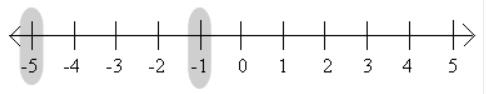Courses

Test: Integers

10 Questions MCQ Test Mathematics (Maths) Class 7 | Test: Integers

Description
Attempt Test: Integers | 10 questions in 10 minutes | Mock test for Class 7 preparation | Free important questions MCQ to study Mathematics (Maths) Class 7 for Class 7 Exam | Download free PDF with solutions
QUESTION: 1

Which integer is greater than –5?

Solution:

An integer on the horizontal number line is greater than the number on its left and less than the number on its right.
Therefore, here -1 will be greater than -5.QUESTION: 2

If a x (b − c) is 8 for a = 2, b = 10 and c = 6 then a x b − a x c is equal to:

Solution:

By Distributive law,
a x (b − c) = a x b - a x c
⇒ a x b - a x c = 8

Alternative method
a x b - a x c = 2 x 10 - 2 x 6
⇒ a x b - a x c = 20 - 12 = 8 [using BODMAS]

Thus, option D is the correct.

QUESTION: 3

The product of three negative integers is:

Solution:

The product of 3 negative integers will always be negative.
a * (-a) = -a2
(-a) * (-a) = a2
(-a) * (-a) * (-a) = a2 * (-a) = -a3

QUESTION: 4

The additive identity for integers is:

Solution:

The additive identity property says that if you add an integer to zero or add zero to the integer, then you get the same integer back i.e. a + 0 = 0 + a = a.

Thus, 0 is an additive identity for the integers.

QUESTION: 5

According to Distributive Law of Multiplication over Addition, a x (b + c) must be equal to:

Solution:

According to Distributive Law of Multiplication over Addition:
a x (b + c) = a x b + a x c

QUESTION: 6

Which amongst the following is the largest?
I-89I, -89, -21, I-21I

Solution:

|x| gives the absolute value of x i.e. the magnitude of the real number without regard to its sign.
The magnitude of a number is its size.

Therefore, I-89I = 89, I-21I = 21

Thus, option C is correct.

QUESTION: 7

The multiplicative identity for integers is:

Solution:

The multiplicative identity of any integer p is a number q which when multiplied with p, leaves it unchanged, i.e. p × q = p.

Since, p × 1 = p = 1 × p
Therefore, 1 is the multiplicative identity for integers.

QUESTION: 8

What will be the additive inverse of 7?

Solution:

The additive inverse of a number x is the number that, when added to x, gives zero i.e. x + (-x) = 0.
This number is also known as the opposite number.

Thus, -7 is the additive inverse of 7.

QUESTION: 9

How many integers are there between -8 and 2 (excluding -8 and 2)?

Solution:

The integers between -8 & 2 are: -7 , -6 , -5 , -4 , -3 , -2 , -1 , 0 , 1

⇒ There are 9 integers between -8 & 2.

QUESTION: 10

The value of [(-4) x (-9) x (-25)] ÷ [(-2) x (-3) x (-5)] is:

Solution:

Firstly we will solve brackets according to BODMAS rule,
(-4) x (-9) x (-25) = -900
(-2) x (-3) x (-5) = -30

⇒ [(-4) x (-9) x (-25)] ÷ [(-2) x (-3) x (-5)]  = (-900)/(-30) = 30Use Code STAYHOME200 and get INR 200 additional OFF Use Coupon Code

Track your progress, build streaks, highlight & save important lessons and more!

Similar ContentRelated tests